Trapezoid v3

The isosceles trapezoid has the angle at a firsst base 40 degrees less than the angle at second base. How large angles has this trapezoid ?

Result

a =  70 °
b =  110 °
c =  110 °
d =  70 °

Solution:

a=d
b=c
a+b+c+d = 360
a+40 = b

a-d = 0
b-c = 0
a+b+c+d = 360
a-b = -40

a = 70
b = 110
c = 110
d = 70

Calculated by our linear equations calculator.

Leave us a comment of this math problem and its solution (i.e. if it is still somewhat unclear...):Be the first to comment!To solve this verbal math problem are needed these knowledge from mathematics:

Do you have a linear equation or system of equations and looking for its solution? Or do you have quadratic equation?

Next similar math problems:

1. Isosceles trapezoid v3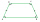In an isosceles trapezoid ABCD is the size of the angle β = 81° Determine size of angles α, γ and δ.
2. IS trapezoid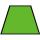Isosceles trapezoid arm measured 35 cm. Height is 30 cm and middle segment is 65 cm. Determine length of its bases.
3. Supplementary angles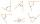One of the supplementary angles are three times larger than the other. What size is larger of supplementary angles?
4. Obtuse angleWhich obtuse angle is creating clocks at 17:00?Number 118 divide into two addends, so first addend is 69 greater than 75% of the second addend.
6. Apples 2How many apples were originally on the tree, if the first day fell one third, the second day quarter of the rest and on tree remained 45 apples?
7. Heating plant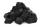Workers dump imported coke at heating plant. On the first day used half of the amount, the second day three quarters the rest and on the third day left 120 tons. How much coke they dump at heating plant?
8. Fifth of the numberThe fifth of the number is by 24 less than that number. What is the number?
9. Unknown numberIdentify unknown number which 1/5 is 40 greater than one tenth of that number.
10. Tickets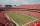On the football tournament ticket cost 45 Kc for standing and 120kč for sitting. Sitting spectators was 1/3 more than standing. The organizers collected a total 12 300 Kc. How many seated and standing seats (spectators)?
11. CandyPeter had a sachet of candy. He wanted to share with his friends. If he gave them 30 candies, he would have 62 candies. If he gave them 40 candies, he would miss 8 candies. How many friends did Peter have?
12. EquationSolve the equation: 1/2-2/8 = 1/10; Write the result as a decimal number.
13. Simple equationSolve for x: 3(x + 2) = x - 18
14. Percentage reduction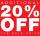Reducing the unknown number by 19% get number 700.7 Determine unknown number.
15. Bakery and flour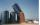The bakery tray for flour was filled to 3/4 volume. After removing 875 kg of flour, it was filled to only 2/5 of the volume. How many tons of flour is in the full tray?
16. Vacation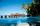Smiths paid a deposit for a vacation of two-sevenths of the total price of the vacation. Then paid also € 550. How much cost their vacation?
17. MeatHalf a kilogram of pork and three-quarters of a kilogram of beef cost total 5.1 USD. One quarter of a kilogram of beef and 1 kilogram of pork cost 4.9 USD. Determine the price of 1 kg of each type of meat.